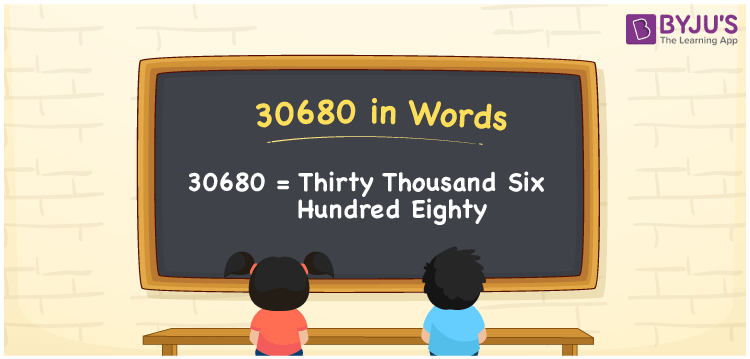# 30680 in Words

30680 in words is Thirty Thousand Six Hundred Eighty. We can convert the number into words with the help of a place value chart easily. For example, if you donated Rs. 30680 to an orphanage home, you can say, “I donated Rupees Thirty Thousand Six Hundred Eighty to an orphanage home”. 30680 is a cardinal number as it depicts a specific quantity. In this article, let us learn the technique of writing number 30680 in word form.

 30680 in Words Thirty Thousand Six Hundred Eighty Thirty Thousand Six Hundred Eighty in numerical form 30680

## 30680 in English Words

We can write numbers in words with the help of the English alphabet. Hence, in English, we can read 30680 as Thirty Thousand Six Hundred Eighty.## How to Write 30680 in Words?

To convert numbers in word form, we use a place value chart. Let us make a chart of 5 columns as 30680 is a five-digit number. The place value chart for the number 30680 is shown below.

 Ten-Thousands Thousands Hundreds Tens Ones 3 0 6 8 0

Thus, we can write the expanded form as:

3 x Ten Thousand + 0 x Thousand + 6 x Hundred + 8 x Ten + 0 x One

= 3 x 10000 + 0 x 1000 + 6 x 100 + 8 x 10 + 0 x 1

= 30000 + 0 + 600 + 80 + 0

= 30000 + 600 + 80

= 30680

= Thirty Thousand Six Hundred Eighty

Hence, 30680 in words is written as Thirty Thousand Six Hundred Eighty

Interesting way of writing 30680 in words

3 = Three

30 = Thirty

306 = Three Hundred and Six

3068 = Three Thousand Sixty-Eight

30680 = Thirty Thousand Six Hundred Eighty

Hence, the word form of the number 30680 is Thirty Thousand Six Hundred Eighty.

30680 is a natural number that precedes 30681 and succeeds 30679

• 30680 in words – Thirty Thousand Six Hundred Eighty
• Is 30680 an odd number? – No
• Is 30680 an even number? – Yes
• Is 30680 a perfect square number? – No
• Is 30680 a perfect cube number? – No
• Is 30680 a prime number? – No
• Is 30680 a composite number? – Yes

## Frequently Asked Questions on 30680 in Words

Q1

### How to write 30680 in words?

30680 in words is written as Thirty Thousand Six Hundred Eighty.
Q2

### Simplify 20000 + 10680, and express in words.

Simplifying 20000 + 10680, we get 30680. Thus, 30680 in words is written as Thirty Thousand Six Hundred Eighty.
Q3

### Is 30680 is an even number?

Yes, 30680 is an even number.